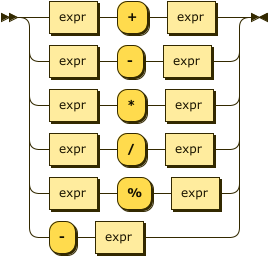A newer version of this documentation is available.

# Arithmetic Operators

• reference
+

Arithmetic operations perform the basic mathematical operations of addition, subtraction, multiplication, division, and modulo within an expression or any numerical value retrieved as part of query clauses. Additionally, N1QL provides a negation operation which changes the sign of a value.

 These arithmetic operators only operate on numbers. In N1QL, arithmetic operators have their usual meaning. However, in any of these expressions: If any operand is MISSING, the value of the expression is MISSING. If any operand is NULL and no operand is MISSING, the value of the expression is NULL. If any operand is not a number, the operator evaluates to NULL.
Operator Description

+

-

Subtract right value from left value.

*

Multiply values.

/

Divide left value by right value.

%

Modulo. Divide left value by right value and return the remainder.

NOTE: Modulo is an integer operator and will use only the integer part of each value.

-`value`

Negate value.

## Syntax

There are six different arithmetic syntaxes:

```expr1 + expr2

expr1 - expr2

expr1 * expr2

expr1 / expr2

expr1 % expr2

-expr1```## Arguments

expr1, expr2

Number or an expression that results in a number value.

Return Values

A number, representing the value of the arithmetic operation.

## Examples

Example 1. Select the longest flight and return its two airports and the distance in feet
Query
``````SELECT sourceairport, destinationairport, ROUND(distance) AS DistanceInMiles,
ROUND(distance)*5280 AS DistanceInFeet
FROM `travel-sample`
WHERE type="route"
ORDER BY distance DESC
LIMIT 1;``````
Returns
``````[
{
"DistanceInFeet": 72906240,
"DistanceInMiles": 13808,
"destinationairport": "DFW",
"sourceairport": "SYD"
}
]``````
Example 2. Select the modulo of 5 and 3 and compare to the modulo of 5.4 and 3.4
Modulo with integers
``SELECT 5 % 3;``
Returns
``````[
{
"\$1": 2
}
]``````
Modulo with fractions
``SELECT 5.4 % 3.4;``
Returns
``````[
{
"\$1": 2
}
]``````

Refer to Comparison Operators for numeric comparisons.

Output